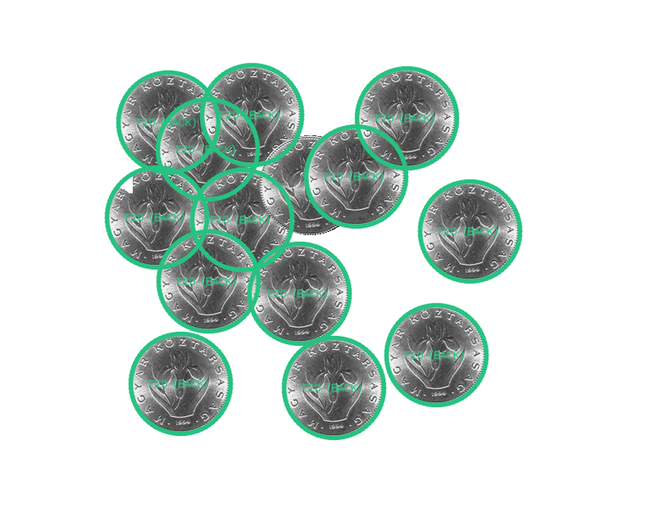# Counting Money

This post is based on a question from the Challenge 24 competition in 2016 where we were given a photograph of each of the denominations of Hungarian currency, called Forints. In addition given a number of photos of piles of coins (some of them counterfeit) and we had to compute the total value of money automatically.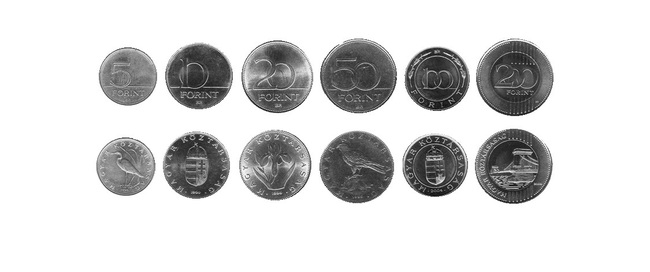First let’s look at the template and see how we can easily extract the location of the clean coins. A flood fill can compute the connected components using a BFS which runs quickly enough and since the images is quite clear we can just iterate through each unvisited non-white pixel and for each start a new component and flood out to all connected pixels that aren’t white. Here’s the code:

``````import cv2
from itertools import product

def flood_fill(img):

rows, cols = img.shape

components = {}
component_id = -1
seen = set()

for (r, c) in product(range(rows), range(cols)):
if inside(r, c, img, seen):

component_id += 1
components[component_id] = []
q = [(r, c)]

while len(q) != 0:

cr, cc = q.pop()
components[component_id].append((cr, cc))

for (dr, dc) in product([-1, 0, 1], repeat=2):

nr, nc = cr + dr, cc + dc

if inside(nr, nc, img, seen):
q.append((nr, nc))
return components
``````

The results look good and it cleanly picks out the backs and fronts of each coin in the template: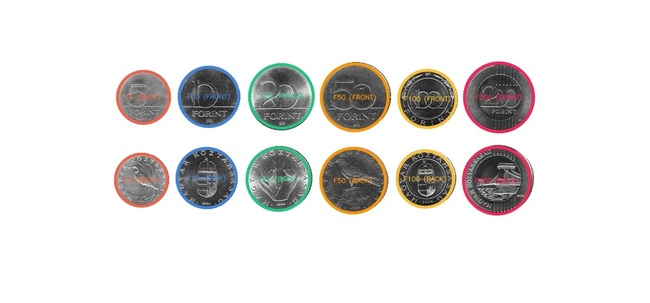Now that we have all the templates we need to find matches of them in images like this, which we will call background images: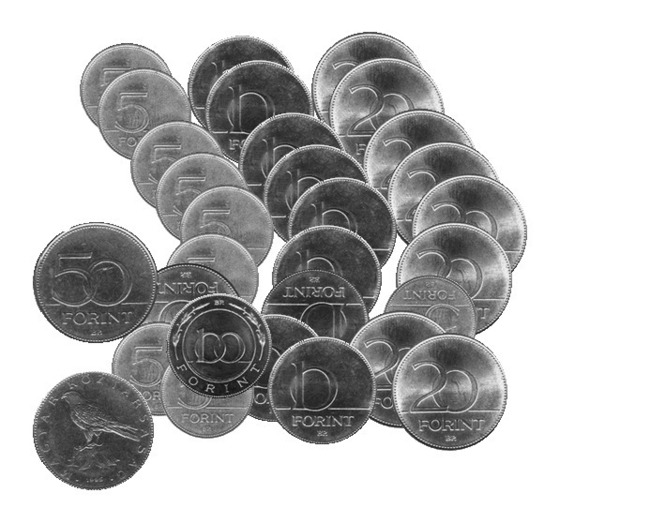Matching coins in images is often presented as an example of image processing algorithms in particular segmentation and the watershed algorithm. There are a couple of things that make this deceptively difficult in this case though. The first is that we aren’t just detecting or counting the coins we actually need to know which denomination each one is so we can total the amount. The second is that the coins can occlude one another so you may only see part of a coin. Finally the coins can each be arbitrarily rotated and fakes coins can appear that are larger or smaller than the originals - these need to be discounted.

There are a few ways of matching templates in an image. One way is to look at the cross-correlation of the two images at different points. You can think of this as sliding the template image row by row, column by column over the background image and at each location measuring how much the two images correlate. So we are looking for the offset that corresponds to the maximum correlation. The problem with this method is that it is super slow especially if the images are large or (like in this case) there are multiple templates to match and we are looking for the best match. We can solve this much faster in the frequency-domain using something called phase correlation. This is the same cross-correlation technical but also isolates the phase information. If `Ga` and `Gb` are the Fourier transforms of the template and background images respectively and `Ga*` and `Gb*` are their copmlex conjugates we can compute this as: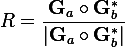and retrieve a normalized (important because there are multiple templates to match) cross-correlation by taking the real component of the inverse Fourier transform of this. Here’s some code that computes the location of the peak of the phase correlation which corresponds to the translation by which the template is off the background image. This process is called image registration.

``````def find_translation(background, template):
from numpy import fft2, ifft2, real, abs, where

br, bc = background.shape
tr, tc = template.shape

Ga = fft2(background)
Gb = fft2(template, (br, bc))

R = Ga * conj(Gb)

pc = ifft2(R / abs(R))
pc = real(pc)

peak = pc.max()
translation = where( pc == peak)

return translation
``````

Running phase correlation for each template iteratively and selecting the highest peak seems to perform well and we are able to correctly register all the coins both back and front in addition to the occluded coins: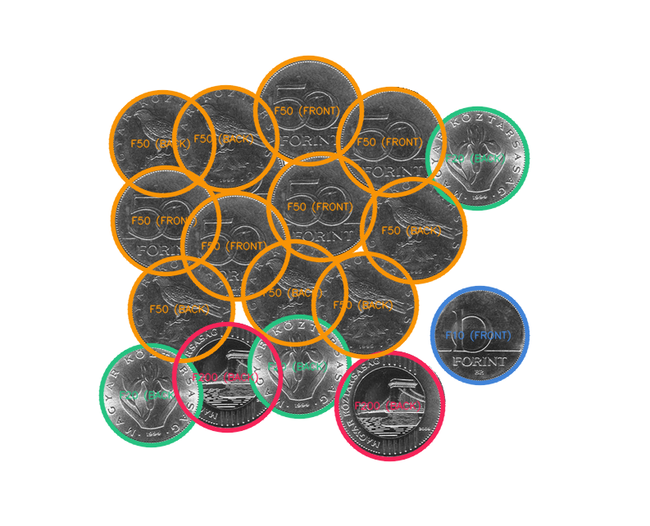This seems to work pretty well and picks out the images however it can’t handle coins that are scaled or rotated. Rotation is actually quite a complex operation if you look at the movement of pixels. Let’s try rearranging the pixels in the image in such a such that they change more predictably when the image is scaled and rotated. We can use some kind of conformal mapping. These are functions that preserve angles in the Euclidean plane and one of the most common is the log-polar transform. Here’s a basic implementation of the log-polar transform:

``````def log_polar(image):
from scipy.ndimage.interpolation import map_coordinates

r, c = image.shape
coords = scipy.mgrid[0:max(image.shape)*2,0:360]

log_r = 10**(coords[0,:]/(r*2.)*log10(c))
angle = 2.*pi*(coords[1,:]/360.)
center = (log_r*cos(angle)+r/2.,log_r*sin(angle)+c/2.)

ret = map_coordinates(image,center,order=3,mode='constant')
return cv2.resize(ret, image.shape)
``````

Here are the resulting log-polar transforms of a few images as they rotate. What’s useful to note here is what happens to horizontal, vertical and radial lines.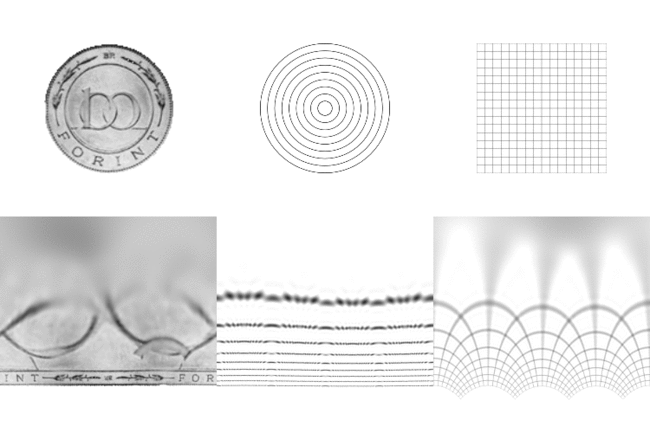So rotation in the log-polar space manifests as a cyclic shift of the columns of pixels. This makes sense because the units of the `X` axis in the images is no longer `X` but the angle of rotation. So pixels on the same angle in the original image (from some center) map to a horizontal line in log-polar space. Similarly pixels on the same radial are mapped to vertical lines `Y`. Another interesting point about this transform is the use of the logarithm. This effectively squashes the bottom rows of the transformed images. Look at the number of pixels dedicated to the text “Forint” compared to the number of pixels dedicated to the center of the images. This mimics the function of the fovea in the human eye and dedicates more resolution to the area in focus which is useful in a number of images tracking applications.

So once we have located the template in the background image we can can use the Fourier shift theorem which tells us that a linear shift in phase manifests as cyclic shift in the Fourier domain. Now we have a way to register two images and compute the translation, rotation and scale factor between them using the following function. Using this information we can detect and count all coins in the image (including the rotated ones) and discount coins that aren’t an authentic size. There are limitations to this method though for example because of the symmetry of the Fourier transform we can only detect a limited range and resolution of scale and rotation. There are more complicate methods that extend it though but thankfully their weren’t needed in this case.

Image registration using spectral methods is really fast and commonly used to align where there is known to be an affine transform between the images. More complex methods are needed to where there is a perspective transform between the two images which will be the topic of an upcoming blog post.